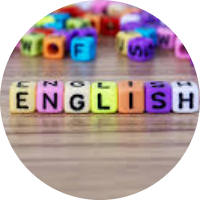# Study of Conic Sections

In this set of MCQs, we shall learn the basics and study some other curves, viz., circles, ellipses, parabolas, and hyperbolas. We will also see how the intersection of a plane with a double-napped right circular cone results in different types of curves.

Start Quiz

Find the equation of circle with center at origin and radius 5 units.

x2+y2=25 x2+y2=5 x2=25 y2=25

Find the equation of circle with center at (2, 5) and radius 5 units.

x2+y2+4x-10y+4=0 x2+y2-4x-10y+4=0 x2+y2+4x+10y+4=0 x2+y2+4x-10y-4=0

Find the center of the circle with equation x2+y2-4x-10y+4=0.

(-2, 5) (-2, -5) (2, -5) (2, 5)

Find the radius of the circle with equation x2+y2-4x-10y+4=0.

25 units 20 units 5 units 10 units

Find the equation of circle which pass through (5, 9) and center at (2, 5).

x2+y2+4x-10y+4=0 x2+y2-4x-10y+4=0 x2+y2+4x+10y+4=0 x2+y2+4x-10y-4=0

At what point of the parabola x² = 9y is the abscissa three times that of ordinate,

(1, 1)

(3, 1)

(-3, 1)

(-3, -3)

In an ellipse, the distance between its foci is 6 and its minor axis is 8 then, its eccentricity is

4/5

1/√52

3/5

1/2

The number of tangents that can be drawn from (1, 2) to x² + y² = 5 is,

0

1

2

More than 2

The perpendicular distance from the point (3, -4) to the line 3x – 4y + 10 = 0 is,

7

8

9

10

The parametric coordinate of any point of the parabola y² = 4ax is,

(-at², -2at)

(-at², 2at)

(a sin²t, -2a sin t)

(a sin t, -2a sin t)

Quiz/Test Summary
Title: Study of Conic Sections
Questions: 10
Contributed by: